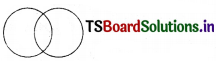# TS 6th Class Maths Bits Chapter 13 Practical Geometry

Solving these TS 6th Class Maths Bits with Answers 13th Lesson Practical Geometry Bits for 10th Class will help students to build their problem-solving skills.

## TS 6th Class Maths Bits Chapter 13 Practical Geometry

Choose the correct answer and write it in the brackets.

Question 1.
$$\overline{\mathrm{AB}}$$ = 4.6 cm. $$\overline{\mathrm{MN}}$$ is constructed such that the length of $$\overline{\mathrm{MN}}$$ is twice that of $$\overline{\mathrm{AB}}$$. Then the length of $$\overline{\mathrm{MN}}$$ is
A) 13.8 cm
B) 9.2 cm
C) 2.3 cm
D) 6.4 cm
B) 9.2 cm

Question 2.
Line segment AB is denoted by
A) $$\overline{\mathrm{AB}}$$
B) $$\overrightarrow{\mathrm{AB}}$$
C) $$\stackrel{\leftrightarrow}{\mathrm{AB}}$$
D) AB
A) $$\overline{\mathrm{AB}}$$

Question 3.
A line segment has _______ end points.
A) 0
B) 1
C) 2
D) 3
C) 2Question 4.
$$\overline{\mathrm{AB}}$$ = 9 cm ’P’ is the mid point of $$\overline{\mathrm{AB}}$$ then $$\overline{\mathrm{AP}}$$ = _______ cm.
A) 13.5
B) 4.5
C) 4
D) 5
B) 4.5

Question 5.
If the diameter of a circle 10 cm then its radius is _______ cms.
A) 4.5
B) 5.5
C) 20
D) 5
D) 5

Question 6.
A circle divides the plane into _______ parts.
A) 1
B) 2
C) 3
D) 4
C) 3Question 7.
From the given figure which points lie inside the circle ______.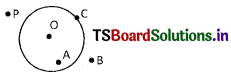A) O, A
B) C
C) B
D) B, P
A) O, A

Question 8.
From the above the points which lie on the circle
A) O, A
B) C
C) B
D) P
B) C

Question 9.
From the given figure $$\overline{\mathbf{x y}}-\overline{\mathbf{z w}}$$ = ______?A) 3 cm
B) 4 cm
C) 4 cm
D) 5 cm
D) 5 cmQuestion 10.
From the above sum $$2 \overline{x y}+\overline{w y}-3 \overline{x z}$$ = ?
A) 9 cm
B) 11 cm
C) 12cm
D) 10 cm
B) 11 cm

Question 11.
What is the angular bisector of 90° ?
A) 45°
B) 60°
C) 50°
D) 55°
A) 45°

Question 12.
Protractor is used to measure _______.
A) lengths of line segments
B) angles
C) to make equal line segments
D) to mark points on a line
B) anglesQuestion 13.
Any two circles can be constructed as follows ?
A) concentric circles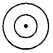B) Intersecting circles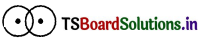C) Circles touch each other externally at a point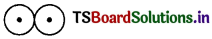D) All the above
D) All the above

Question 14.
Statement (X) : If two circles are congruent their radii are equal
Statement (Y): Length of a line segment is measured by a ruler
Statement (Z): If two line segments are equal then their lengths are need not to be equal
A) X, Y, Z are true
B) X is true Y is false, Z is true
C) X is false, Y is true, Z is false
D) X, Y are true, Z is false
D) X, Y are true, Z is false

Question 15.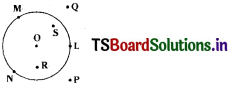Statement (P) : Points O, S, R are lie inside the circle
Statement (Q): points M, N, L are on the circle
Statement (R) : Q, P points lie outside the circle
A) P, Q, R are true
B) P is true, Q is false, R is true
C) P, Q are true, R is false
D) P is true, Q, R are false
A) P, Q, R are trueQuestion 16.From the adjacent figure $$\overline{\mathbf{O N}}$$ is the angular bisector of ∠LOM
Statement (L) : ∠LON = ∠MON
Statement (M) : 1/2 ∠MON = ∠LOM
Statement (N): ∠LON + ∠MON = ∠LOM
A) L, M and N are true
B) L is true M is false, N is true
C) L and M are, N is false
D) L and are false, N is true
B) L is true M is false, N is true

Question 17.
Construction of 40° with theprotractor
Step (1) : Mark a point P at 40°
Step (2) : Place the centre point of the protractor at Q and the line aligned with $$\overline{\mathrm{QR}}$$.
Step (3): Joint QP. ∠RPQ is the required angle
Step (4) : Draw a ray of an length $$\overline{\mathrm{QR}}$$.
A) (4) – (2) – (1) – (3)
B) (4) – (3) – (2) – (1)
C) (4) – (1) – (2) – (3)
D) (4) – (2) – (3) – (1)
A) (4) – (2) – (1) – (3)

Question 18.
Construction to bisect a given angle ∠MON
Step (1) : With P as centre draw any radius more than half of PQ in the interior of ∠MON
Step (2) : With Q as centre without altering radius draw another arc in the interior of ∠MON
Step (3) : With ’O’ as centre and any convenient radius, draw an arc $$\overparen{P Q}$$ cutting OM and ON at P and Q resp.
Step (4): Draw ray $$\overline{\mathrm{OZ}}$$. Then $$\overline{\mathrm{OZ}}$$ is the designed bisector of ∠MON
∴ ∠MOZ = ∠ZON
A) (1) – (2) – (3) – (4)
B) (1) – (3) – (2) – (4)
C) (3) – (1) – (2) – (4)
D) (3) – (2) – (1) – (4)
C) (3) – (1) – (2) – (4)Question 19.
Which of the following instrument is not used to construct the shapes ?
A) the compass
B) the divider
C) the protractor
D) barometer
D) barometer

Question 20.
Using ruler and compass which of the following construction can be made ?
A) A circle, with given radius
B) A line segment, with given length
C) Perpendicular bisector of a line segment
D) All the above
D) All the above

Question 21.
The line segment MN is denoted by
A) $$\stackrel{\leftrightarrow}{\mathrm{MN}}$$
B) $$\overrightarrow{\mathrm{MN}}$$
C) $$\overleftarrow{\mathrm{MN}}$$
D) $$\overline{\mathrm{MN}}$$
D) $$\overline{\mathrm{MN}}$$Question 22.
The instrument used to measure an angle is a
A) Protractor
B) Compasses
C) Ruler
D) Divider
A) Protractor

Question 23.
The instrument used to measure a line segment is
A) Compasses
B) Divider
C) Ruler
D) Protractor
C) Ruler

Question 24.
The instrument used to mark points on a line is a
A) Protractor
B) Divider
C) Compasses
D) Ruler
B) DividerQuestion 25.
Which of the following is a divider ?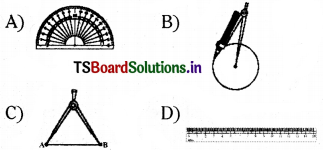C)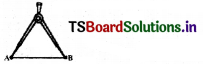Question 26.
Which of the following is a protractor?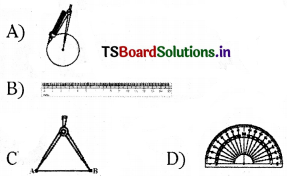D)Question 27.
Which of the following is a compass?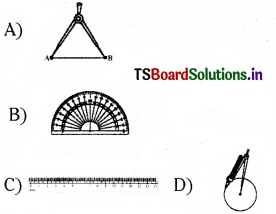D)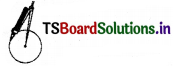Question 28.
Which of the following circles intersect at two points ?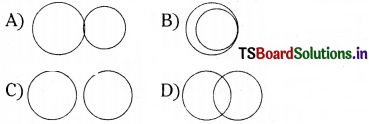D)